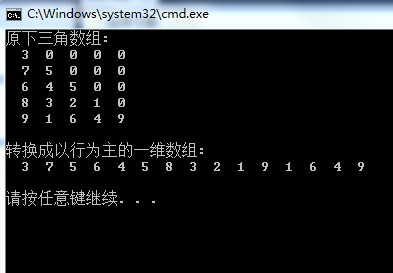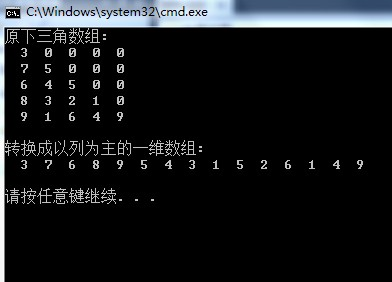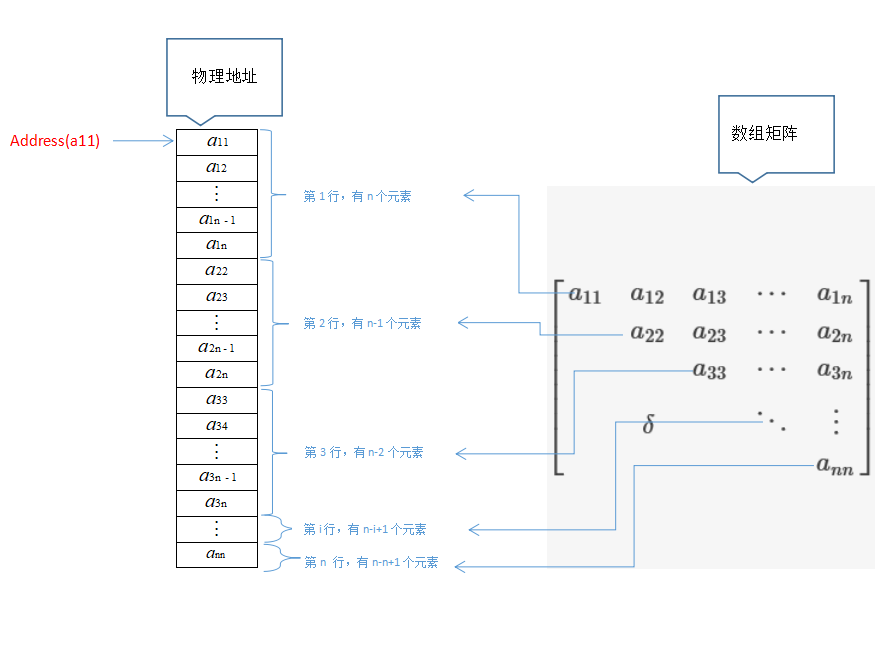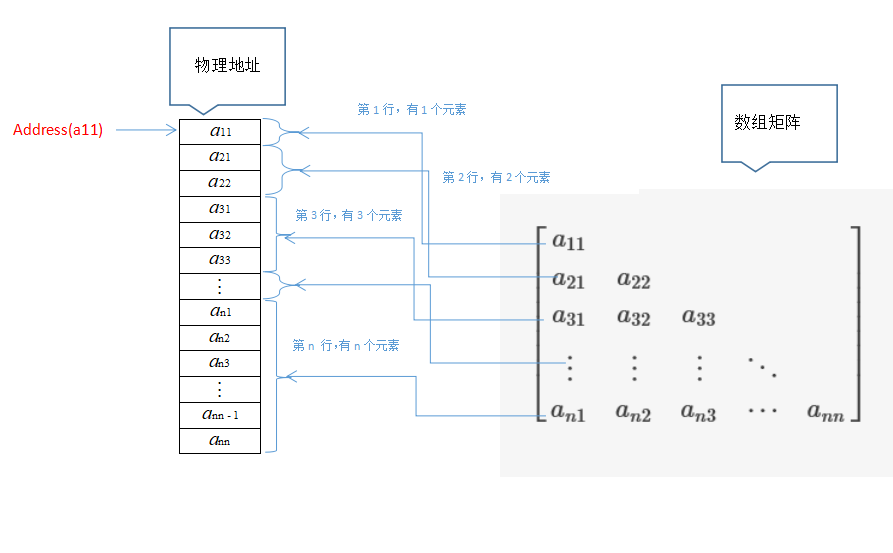• 要求编写算法把矩阵A采用压缩存储存储一维数组B， 并且依次输出B各元素的值以验证该算法功能已实现 */ #include using namespace std; const int m=5; const int n=5; const int c=16; int B[c]; //...
/*1.  已知矩阵A是一个下三角矩阵，如下图
要求编写算法把矩阵A采用压缩存储，存储到一维数组B中，
并且依次输出B中各元素的值以验证该算法功能已实现
*/
#include<iostream>
using namespace std;
const int m=5;
const int n=5;
const int c=16;
int B[c];               //定义一维数组B,长度为16
//初始化数组A
int A[m][n]={{1},{4,7},{6,9,5},{1,8,4,1},{2,3,0,9,6}};
int main()
{
int i,j;
int k;
cout<<"输出二维数组的三角矩阵："<<endl;
for(i=0;i<m;i++)  //输出二维数组A的矩阵
{
for(j=0;j<n;j++)
cout<<A[i][j]<<" ";
cout<<endl;
}
cout<<endl;
//对三角矩阵进行压缩
for(i=0;i<m;i++)                //遍历三角矩阵
{
for(j=0;j<n;j++)
if(i>=j)				//如果行号大于等于列号
{
k=i*(i+1)/2+j;		//求出各元素的下标

B[k]=A[i][j];		//存储元素

}
else					//如果行号小于列号
{
k=c-1;				//将元素下标定为c-1
B[k]=0;				//元素的值存储为0
}

}
cout<<"输出三角矩阵压缩后的一维矩阵："<<endl;
for(k=0;k<c;k++)					//按顺序输出压缩后的元素
cout<<B[k]<<" ";
cout<<endl;
system("pause");
return 0;
}



展开全文算法 system c
• (1) 将大小为n*n的下三角数组转换成以行为主的一维数组，且不存储内容为0的元素！ data[i][j]的位置 =i*(i+1)/2+j;   (2) 将大小为n*n的下三角数组转换成以列为主的一维数组，且不存储内容为0的元素！ data[i][j]...
(1) 将大小为n*n的下三角数组转换成以行为主的一维数组，且不存储内容为0的元素！
data[i][j]的位置 =i*(i+1)/2+j;

(2) 将大小为n*n的下三角数组转换成以列为主的一维数组，且不存储内容为0的元素！
data[i][j]的位置=[n+(n-j+1)]*j/2+(i-j)

(1)#include <iostream> #include <iomanip> using namespace std;
int Lower={  3,0,0,0,0,  7,5,0,0,0,  6,4,5,0,0,  8,3,2,1,0,  9,1,6,4,9 };

int main() {  int RowMajor;  int Index,i,j;

cout<<"原下三角数组:\n";  for(i=0;i<5;i++)  {   for(j=0;j<5;j++)    cout<<setw(3)<<Lower[i][j];   cout<<endl;  }

//进行以行为主的数组数据转换  for(i=0;i<5;i++)   for(j=0;j<5;j++)    if(i>=j)    {     Index=i*(i+1)/2+j;     RowMajor[Index]=Lower[i][j];    }  cout<<endl;

cout<<"转换成以行为主的一维数组:\n";  for(i=0;i<15;i++)   cout<<setw(3)<<RowMajor[i];  cout<<"\n\n";
return 0; }

(2)#include <iostream> #include <iomanip> using namespace std;
int Lower={  3,0,0,0,0,  7,5,0,0,0,  6,4,5,0,0,  8,3,2,1,0,  9,1,6,4,9 };

int main() {  int ColMajor;  int Index,i,j;
cout<<"原下三角数组:\n";  for(i=0;i<5;i++)  {   for(j=0;j<5;j++)    cout<<setw(3)<<Lower[i][j];   cout<<endl;  }

//进行以列为主的数组数据转换  for(i=0;i<5;i++)   for(j=0;j<5;j++)    if(i>=j)    {     Index=(11-j)*j/2+(i-j);     ColMajor[Index]=Lower[i][j];    }  cout<<endl;

cout<<"转换成以列为主的一维数组:\n";  for(i=0;i<15;i++)   cout<<setw(3)<<ColMajor[i];  cout<<"\n\n";
return 0; }


展开全文• 一维数组的地址计算 设每个元素的大小是size，首元素的地址是a，则 a[i] = a + (i-1)*size 若首元素的地址是a 则a[i] = a + i*size 二维数组的地址计算 (m*n的矩阵) 行优先 设每个元素的大小是size，首...
一维数组的地址计算 设每个元素的大小是size，首元素的地址是a，则 a[i] = a + (i-1)*size
若首元素的地址是a 则a[i] = a + i*size
二维数组的地址计算 (m*n的矩阵) 行优先 设每个元素的大小是size，首元素的地址是a，则a[i][j]? 分析：a[i][j]位于第i行，第j列。它之前有i-1行，在第i行它之前有j-1个元素。 即a[i][j] = a + [n*(i-1) + (j-1)]*size
三维数组的地址计算 (rmn) r行m列n纵 行优先 首元素的地址a[1,1,1]
a[i,j,k] = a[1,1,1] + [(i-1)*n*m + (j-1)*n + (k-1)]*size
压缩存储：指为多个值相同的元素只分配一个存储空间，对零元素不分配存储空间，其目的是为了节省存储空间。
二维数组通常用来存储矩阵，特殊矩阵分为两类： （1）元素分布没有规律的矩阵，按照规律对用的公式实现压缩。 （2）无规律，但非零元素很少的稀疏矩阵，只存储非零元素实现压缩。
一、三角矩阵 包括上三角矩阵，下三角矩阵和对称矩阵 (1)若i<j时，ai,j=0，则称此矩阵为下三角矩阵。 (2)若i>j时，ai,j=0，则称此矩阵为上三角矩阵。 (3)若矩阵中的所有元素满足ai,j=aj,i，则称此矩阵为对称矩阵。 下三角上三角二、三对角矩阵带状矩阵的压缩方法：将非零元素按照行优先存入一维数组。 （1）确定一维数组的存储空间大小：2+(n-2)*3+2 = 3n-2 （2）确定非零元素在一维数组中的地址 loc(i,j) = loc(1,1) + 前i-1行非零元素个数+第i行中ai,j前非零元素的个数 前i-1行：3 * (i-1) - 1，因为第一行只有两个，所以要减去1 第i行中ai,j前非零元素的个数=(j-i)+1, j-i有三种情况： （1）j<i j-i=-1 （2）j==i j-i=0 （3）j>i j-i=1
loc(i,j) = loc(1,1) + 3(i-1)-1 + j-i+1 = loc(1,1) + 2(i-1) + j-1 = loc(1,1) + 2i+j-3
展开全文• 数据结构-二维数组-三角矩阵压缩存储一、什么是三角矩阵前情提要三角矩阵也是属于类特殊的二维数组矩阵，同样也用压缩的存储方式，能够更好的节约存储空间，二维数组三角矩阵分为上三角矩阵下三角矩阵，其实现...
数据结构-二维数组-三角矩阵压缩存储
一、什么是三角矩阵
前情提要
三角矩阵也是属于一类特殊的二维数组矩阵，同样也用压缩的存储方式，能够更好的节约存储空间，二维数组的三角矩阵分为上三角矩阵和下三角矩阵，其实现的原理差不多类似，下面就细细道来。
三角矩阵的特点
此处讨论的三角矩阵的行数和列数是一样的，不妨设都设为

n
$n$。如下所示：
⎡⎣⎢⎢⎢⎢⎢⎢⎢⎢⎢⎢⎢⎢a11a12a22a13a23a33δa14a24a34a44⋯⋯⋯⋯⋱a1n−1a2n−1a3n−1a4n−1⋮an−1n−1a1na2na3na4n⋮an−1nann⎤⎦⎥⎥⎥⎥⎥⎥⎥⎥⎥⎥⎥⎥\begin{bmatrix}
{a_{11}}&{a_{12}}&{a_{13}}&{a_{14}}&\cdots&{a_{1n-1}}&{a_{1n}}\\
{}&{a_{22}}&{a_{23}}&{a_{24}}&\cdots&{a_{2n-1}}&{a_{2n}}\\
{}&{}&{a_{33}}&{a_{34}}&\cdots&{a_{3n-1}}&{a_{3n}}\\
{}&{}&{}&{a_{44}}&\cdots&{a_{4n-1}}&{a_{4n}}\\
{}&{}&\delta&{}&\ddots&\vdots&\vdots\\
{}&{}&{}&{}&{}&{a_{n-1n-1}}&{a_{n-1n}}\\
{}&{}&{}&{}&{}&{}&{a_{nn}}
\end{bmatrix}  如上所示，为上三角矩阵，矩阵的对角线以下的所有元素均为同一常数

δ
$\delta$,或者无效的数据。从上往下逐行的元素总数是比上一行少一个，构成等差数列条件，以下会用的等差数列数学知识。若

δ
$\delta$为常数，则需要在所有元素的最后一个另外加一个元素位置单独存放该数据，毕竟只要是有效数据就需要存储的嘛。对于下三角矩阵有类似的特点，这里放到公式推导里面去介绍。
二、三角矩阵压缩存储
上三角矩阵的存储
如下所示：对于元素处于上三角区域，即元素

aij
$a_{ij}$，其中

i≤j
$i \le j$，可得如下规律：  第

1
$1$行有n$n$个元素，第

2
$2$行有(n−1)$(n-1)$个元素，第

3
$3$行有(n−2)$(n-2)$个元素，第

i
$i$行有(n−i+1)$(n-i+1)$个元素，…第

n
$n$行有(n−n+1)$(n-n+1)$(即只有

1
$1$个元素)个元素；可得对于元素aij$a_{ij}$，第

(i−1)
$(i-1)$行（即元素

aij
$a_{ij}$前一行）共有：

∑k=1i−1(n−k+1)=(i−1)(2n−i+2)2

\sum_{k=1}^{i-1}(n-k+1)=\frac {(i-1)(2n-i+2)}{2}个元素，元素

aij
${a_{ij}}$,在第i行中是第

(j−i+1)
$(j-i+1)$个元素，规定：每个元素所占的长度为

e
$e$，所以：address(aij)=address(a11)+((i−1)(2n−i+2)2+j−i)eaddress({a_{ij}})=address({a_{11}})+(\frac {(i-1)(2n-i+2)}{2}+j-i)e
对于元素处于下三角区域，即元素

aij
$a_{ij}$，其中

i>j
$i \gt j$，因为下三角区的元素值都一样（如果元素的值有效），则把它放到存储区的最后一个单元,即：

(n+1)n2+1
$\frac {(n+1)n}{2}+1$的位置，可得地址公式：

下三角矩阵的存储
如下所示：对于元素处于下三角区域，即元素

aij
$a_{ij}$，其中

i≥j
$i \ge j$，可得如下规律：  第

1
$1$行有1$1$个元素，第

2
$2$行有2$2$个元素，第

3
$3$行有3$3$个元素，第

i
$i$行有i$i$个元素，…第

n
$n$行有n$n$个元素；可得对于元素

aij
$a_{ij}$，第

(i−1)
$(i-1)$行（即元素

aij
$a_{ij}$前一行）共有：

∑k=1i−1k=(i−1)(1+i−1)2=(i−1)i2

\sum_{k=1}^{i-1}k=\frac {(i-1)(1+i-1)}{2}=\frac {(i-1)i}{2}个元素,元素

aij
${a_{ij}}$在第

i
$i$行为第j$j$个元素，现规定每个元素占用的单位为

e
$e$，可得：

(n+1)n2+1
$\frac {(n+1)n}{2}+1$的位置，可得地址公式：

矩阵的压缩存储暂时写到这，写得不好，多多指教哈。
展开全文数据结构
• 列主映射:将矩阵依此从每一列的索引从上至连续编号，将二维矩阵的索引映射为一维数组。 本文的多种矩阵均采用行主映射，使用一维数组来表达多种矩阵。例如按照常规对二维矩阵进行描述的C++二维数组矩阵元素M(3...
• LOC（…）表示该坐标对应的存储中的地址（一维坐标） PS.对与简单的二维数组则①求出该数组前面有多少个元素（分清行主序还是列主序）， ②第一个元素对应的地址加上前面所有元素的总字节数 二，对称矩阵 三，上...数据结构 C语言 广义表
•   对于程序n阶对称矩阵，一般可采取压缩算法，即用一维数组存储相同矩阵元素得一个副本. 定义式： 一维存储 n(n+1)/2 个 k= i(i-1)/2+j-1 当i>=j时 k= j(j-1)/2+i-1 当i<j时 *k为一维数组下标 i为行数...映射
• 对于主存储器来说，存储空间十分珍贵，矩阵的表示有几类特殊的矩阵，如：对角矩阵、三对角矩阵、上三角矩阵下三角矩阵、对称矩阵等，其中有大量的重复数据，在存储过程就需要进行压缩处理，使得主存储器的...数据结构
• 维普资讯 http://doc.xuehai.net本目任辑：媛 栏责编谢媛开发研究与设计技术C语言循环语句维数组中的应用从艳，益夫。喜梅任刘(北农业大学海洋学院，北秦皇岛 06 0 )河河 6 03摘要：据循环语句遍历数组元素的...
• 数据结构-二维数组-对称矩阵压缩存储一、什么是对称矩阵对称矩阵，顾名思义就是矩阵中的元素是对称的，那具体是关于什么对称呢？就是关于对角线对策，即关于左上角到右角的对角线对称数据结构
• 输出压缩后的一维数组；根据输入的行号列号，从压缩矩阵中计算出元素的值 #include<stdio.h> int main(){ inta={ //定义原二维数组 1,0, 0, 0, 0, 5,9, 0, 0, 0, 4,6,...
• SWPU-DS）若有 n 阶对称矩阵 A，以行序为主序方式，将其三角形的元素(包括主对角线上所有元素)依次存放于一维数组B[1…(n(n+1))/2]，则 B 确定 a[i, j]（i<j）的位置 k 的关系为（ ）。
• .三角矩阵的概念 以主对角线划分三角矩阵下三角矩阵和上三角矩阵 ...根据上、下三角矩阵的特殊性(有小半部分的元素都为个常数C或者0)我们可以考虑将这一半的空间压缩到个元素（多对的映射)，然后另...
• 但是在矩阵中非零元素呈某种规律分布或者矩阵中出现大量的零元素的情况，看起来存储密度仍为1，但实际上占用了许多单元去存储重复的非零元素或零元素，这对高阶矩阵会造成极大的浪费，为了节省存储空间， 我们可以...
• 处理海量数据时，我把数据经过计算并存在了矩阵中（n×n的二维数组），需要开辟约8000*8000的空间，而且只存成了上三角（数据需要），因此有一半的空间是浪费的（存着无意义的数字0或-1），开辟空间以及数据持久...
• NumPy是Python的最重要的扩展程序库之一，也是...教程内容分为向量（一维数组）、矩阵（二维数组）、三维与更高维数组3个部分。 Numpy数组与Python列表 介绍正式内容之前，先让我们先来了解一下Numpy数组与Pyt..python java 机器学习 人工智能 大数据
• 数组是由类型相同的数据元素构成的有序集合,每个数据元素称为个数组元素,每个元素受(n>=1)个线性关系的的约束,每个元素n个线性关系的序号i1,i2...in称为该元素的下标,并称该数组为n维数组. 数组的存储结构与...数据结构 压缩
• 三角矩阵 三角矩阵的常用压缩方式有两种： 线性压缩 使用三角形的二维数组压缩 线性压缩存储三角矩阵 下三角矩阵： ...上三角矩阵： ...以下三角矩阵的线性压缩存储为例，... * 下三角矩阵线性压缩存储 */ public cl...数据结构
• .三角矩阵的概念 以主对角线划分三角矩阵下三角矩阵和上三角矩阵 下三角矩阵：矩阵(除主对角线)的上...根据上、下三角矩阵的特殊性(有小半部分的元素都为个常数C或者0)我们可以考虑将这一半的空间压缩到...
• 一维数组即为线性表，二维数组可以看作是元素为一维数组的（线性表的）线性表，以此类推n维数组为元素为n-1维数组的线性表。 行主映射和列主映射 数组的应用需要我们将数组元素序列化，即按一维数组排列 。令n是一...
• 源代码：// 4_a1.cpp -- 下三角矩阵的压缩存储定义 /* * -> 题目要求： * 1.已知矩阵A是一个下三角矩阵，如下图 ... * 2.要求编写算法把矩阵A采用压缩存储存储一维数组B;测试 算法 数据结构 delete
• 、PTA实验作业 题目1：7-3 出生年 1. 本题PTA提交列表 2. 设计思路 定义循环变量i,year变化年份,year1出生年份,不同数字n,count不同数字数; 定义数组num,a={0},num放对应数字及其个数,a={0}年份...c/c++ 数据结构与算法
• 声明：文章为博主原创，转载请联系博主。文章若有错误和疏漏之处，还望大家不吝赐教！ 第章：数据结构与算法基础===============================...1.数据结构基础与线性表：下三角矩阵元素存储位置计算、队列的...
• 数组 矩阵 广义表5.1 数组5.1.1 数组的类型5.1.2 定义和表示方法5.1.3 例题5.2 矩阵的压缩存储5.2.1 矩阵5.2.2 特殊矩阵5.2.2.1 对称矩阵例题5.2.2.2 三角矩阵5.2.2.3 三对角矩阵例题5.2.3 稀疏矩阵5.2.3.1 三元组...
• 一维数组 数组名实际上就是数组首地址，是一个地址常量，本质上是一个数 数组名也可以进行运算，是逻辑上的运算，并不是直接的数字运算 arr和*arr是一样的 *(arr+1)和arr是一样的 arr和&arr是一样...数据结构
•   数组(array)是具有相同类型的数据的集合，也就是说数组的所有元素的类型都是相同的，所有的数据结构，数组算是最常见也是最简单的一种数据结构，我们最常见的也就是一维数组，当然还有二维，三维……，数组...数据结构...